# Java Program to copy all elements of one array into another array

In this tutorial you will learn about the Java Program to copy all elements of one array into another array and its application with practical example.

## Java Program to copy all elements of one array into another array

In this tutorial, we will learn to create a Java program that will copy all elements of one array into another array using Java programming.

## Prerequisites

Before starting with this tutorial, we assume that you are the best aware of the following Java programming topics:

• Operators in Java Programming.
• Basic Input and Output function in Java Programming.
• Basic Java programming.
• For loop in Java programming.
• Arithmetic operations in Java Programming.

## Copy all array elements to another array.

In this program, First, we will first declare the size of the array and its elements for the prorgam. Then we will find the length of array 1 using the length function for the second array. After that we will copy the elements of the array one to array two. At last, we will print the first and the second array to the user.

With the help of this program, we can copy all elements of one array into another array.

## Output:-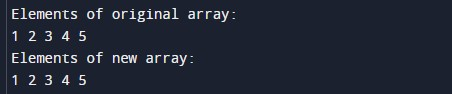In the above program, we have first initialized the required variable.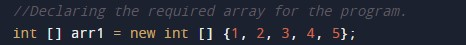• arr1[] = it will hold the integer value.
• arr2[] = it will hold the integer value.
• i = it will hold the integer value.

Finding the size of the array one using the length function.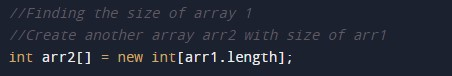Copying the elements of the array one to the second array.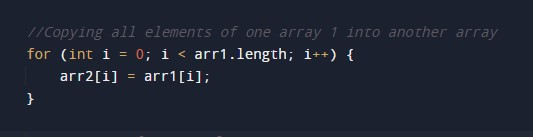Printing array 1 to the user.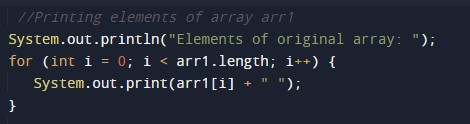Printing array 2 to the user.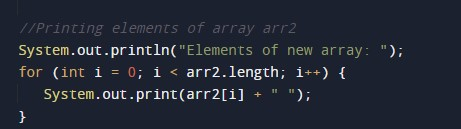In this tutorial we have learn about the Java Program to copy all elements of one array into another array and its application with practical example. I hope you will like this tutorial.